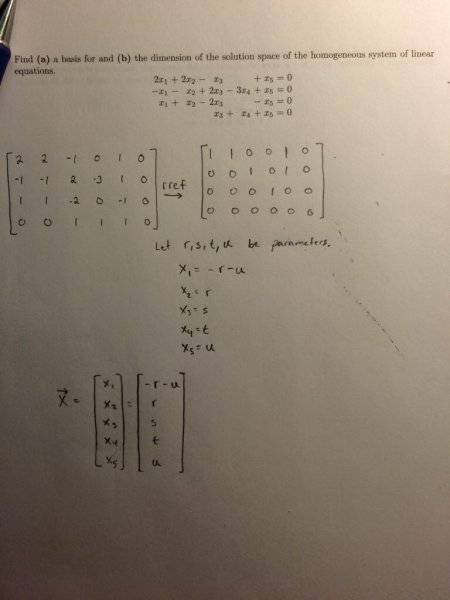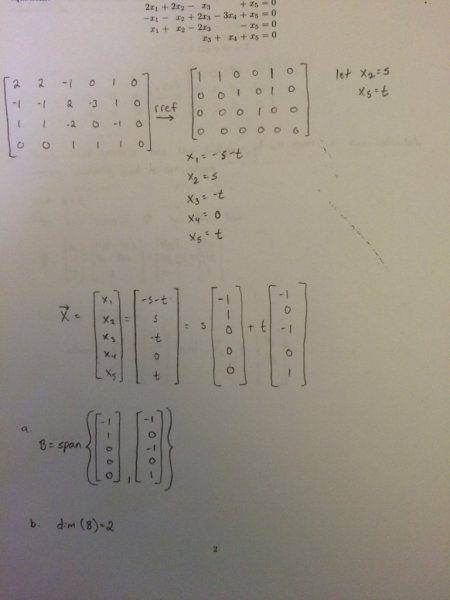# Basis and Dimension of Solution Space

## Homework Statement

Find a basis for and the dimension of the solution space of the homogenous system of equations.

2x1+2x2-x3+x5=0
-x1-x2+2x3-3x4+x5=0
x1+x2-2x3-x5=0
x3+x4+x5=0

## The Attempt at a Solution

I reduced the vector reduced row echelon form. However the second row doesn't have a 1 in the x2 position which has thrown me off. Do I parametrize here like I did? Something just doesn't seem correct.
Here is my work thus far:

Last edited:Mark44
Mentor
Based on your final matrix, which I didn't check, you have
x1 = -x2 - x5
x2 = x2
x3 = -x5
x4 = 0
x5 = x5
If you stare at this long enough you should see that the above represents all possible linear combinations of two vectors.

BTW, it's confusing to have that last column of zeroes in your matrix. It would be better to eliminate that column since it can't possibly change.

Well I got the following equations from the matrix:
x1+x2+x4=0
x3+x5=0
x4=0
Not really sure how I can parametrize these to solve for the basis of the solution space.

HallsofIvy
Homework Helper
If those are correct, since $x_4= 0$, your first equation is $x_1+ x_2= 0$ which means that $x_2= -x_1$. And, of course, your second equation s the same as $x_5= -x_3$. That means we can write $<x_1, x_2, x_3, x_4, x_5>= <x_1, -x_1, x_3, 0, -x_3>= <x_1, -x_1, 0, 0, 0>+ <0, 0, x_3, 0, -x_3>= x_1<1, -1, 0, 0, 0>+ x_3<0, 0, 1, 0, -1>$. Now do you see what basis vectors for the solution space are?

This is the solution I got. However I am a bit confused on my basis. How can the x4 slot both have zeros in the basis vectors and still span the space? Did I make a mistake along the way?
Thanks for the help everyone.Mark44
Mentor
This is the solution I got. However I am a bit confused on my basis. How can the x4 slot both have zeros in the basis vectors and still span the space? Did I make a mistake along the way?
Thanks for the help everyone.

View attachment 80599
There's no reason that the x4 component shouldn't be zero. You can do a quick check by multiplying each of the two vectors by your matrix. If you don't get a zero vector for each product, that's a sign that you did something wrong.

HallsofIvy